# `sklearn.linear_model`.SGDClassifier¶

class `sklearn.linear_model.``SGDClassifier`(loss=’hinge’, penalty=’l2’, alpha=0.0001, l1_ratio=0.15, fit_intercept=True, max_iter=None, tol=None, shuffle=True, verbose=0, epsilon=0.1, n_jobs=1, random_state=None, learning_rate=’optimal’, eta0=0.0, power_t=0.5, class_weight=None, warm_start=False, average=False, n_iter=None)[source]

Linear classifiers (SVM, logistic regression, a.o.) with SGD training.

This estimator implements regularized linear models with stochastic gradient descent (SGD) learning: the gradient of the loss is estimated each sample at a time and the model is updated along the way with a decreasing strength schedule (aka learning rate). SGD allows minibatch (online/out-of-core) learning, see the partial_fit method. For best results using the default learning rate schedule, the data should have zero mean and unit variance.

This implementation works with data represented as dense or sparse arrays of floating point values for the features. The model it fits can be controlled with the loss parameter; by default, it fits a linear support vector machine (SVM).

The regularizer is a penalty added to the loss function that shrinks model parameters towards the zero vector using either the squared euclidean norm L2 or the absolute norm L1 or a combination of both (Elastic Net). If the parameter update crosses the 0.0 value because of the regularizer, the update is truncated to 0.0 to allow for learning sparse models and achieve online feature selection.

Read more in the User Guide.

Parameters: loss : str, default: ‘hinge’ The loss function to be used. Defaults to ‘hinge’, which gives a linear SVM. The possible options are ‘hinge’, ‘log’, ‘modified_huber’, ‘squared_hinge’, ‘perceptron’, or a regression loss: ‘squared_loss’, ‘huber’, ‘epsilon_insensitive’, or ‘squared_epsilon_insensitive’. The ‘log’ loss gives logistic regression, a probabilistic classifier. ‘modified_huber’ is another smooth loss that brings tolerance to outliers as well as probability estimates. ‘squared_hinge’ is like hinge but is quadratically penalized. ‘perceptron’ is the linear loss used by the perceptron algorithm. The other losses are designed for regression but can be useful in classification as well; see SGDRegressor for a description. penalty : str, ‘none’, ‘l2’, ‘l1’, or ‘elasticnet’ The penalty (aka regularization term) to be used. Defaults to ‘l2’ which is the standard regularizer for linear SVM models. ‘l1’ and ‘elasticnet’ might bring sparsity to the model (feature selection) not achievable with ‘l2’. alpha : float Constant that multiplies the regularization term. Defaults to 0.0001 Also used to compute learning_rate when set to ‘optimal’. l1_ratio : float The Elastic Net mixing parameter, with 0 <= l1_ratio <= 1. l1_ratio=0 corresponds to L2 penalty, l1_ratio=1 to L1. Defaults to 0.15. fit_intercept : bool Whether the intercept should be estimated or not. If False, the data is assumed to be already centered. Defaults to True. max_iter : int, optional The maximum number of passes over the training data (aka epochs). It only impacts the behavior in the `fit` method, and not the partial_fit. Defaults to 5. Defaults to 1000 from 0.21, or if tol is not None. New in version 0.19. tol : float or None, optional The stopping criterion. If it is not None, the iterations will stop when (loss > previous_loss - tol). Defaults to None. Defaults to 1e-3 from 0.21. New in version 0.19. shuffle : bool, optional Whether or not the training data should be shuffled after each epoch. Defaults to True. verbose : integer, optional The verbosity level epsilon : float Epsilon in the epsilon-insensitive loss functions; only if loss is ‘huber’, ‘epsilon_insensitive’, or ‘squared_epsilon_insensitive’. For ‘huber’, determines the threshold at which it becomes less important to get the prediction exactly right. For epsilon-insensitive, any differences between the current prediction and the correct label are ignored if they are less than this threshold. n_jobs : integer, optional The number of CPUs to use to do the OVA (One Versus All, for multi-class problems) computation. -1 means ‘all CPUs’. Defaults to 1. random_state : int, RandomState instance or None, optional (default=None) The seed of the pseudo random number generator to use when shuffling the data. If int, random_state is the seed used by the random number generator; If RandomState instance, random_state is the random number generator; If None, the random number generator is the RandomState instance used by np.random. learning_rate : string, optional The learning rate schedule: ‘constant’: eta = eta0 ‘optimal’: eta = 1.0 / (alpha * (t + t0)) [default] ‘invscaling’: eta = eta0 / pow(t, power_t) where t0 is chosen by a heuristic proposed by Leon Bottou. eta0 : double The initial learning rate for the ‘constant’ or ‘invscaling’ schedules. The default value is 0.0 as eta0 is not used by the default schedule ‘optimal’. power_t : double The exponent for inverse scaling learning rate [default 0.5]. class_weight : dict, {class_label: weight} or “balanced” or None, optional Preset for the class_weight fit parameter. Weights associated with classes. If not given, all classes are supposed to have weight one. The “balanced” mode uses the values of y to automatically adjust weights inversely proportional to class frequencies in the input data as `n_samples / (n_classes * np.bincount(y))` warm_start : bool, optional When set to True, reuse the solution of the previous call to fit as initialization, otherwise, just erase the previous solution. average : bool or int, optional When set to True, computes the averaged SGD weights and stores the result in the `coef_` attribute. If set to an int greater than 1, averaging will begin once the total number of samples seen reaches average. So `average=10` will begin averaging after seeing 10 samples. n_iter : int, optional The number of passes over the training data (aka epochs). Defaults to None. Deprecated, will be removed in 0.21. Changed in version 0.19: Deprecated coef_ : array, shape (1, n_features) if n_classes == 2 else (n_classes, n_features) Weights assigned to the features. intercept_ : array, shape (1,) if n_classes == 2 else (n_classes,) Constants in decision function. n_iter_ : int The actual number of iterations to reach the stopping criterion. For multiclass fits, it is the maximum over every binary fit. loss_function_ : concrete `LossFunction`

`LinearSVC`, `LogisticRegression`, `Perceptron`

Examples

```>>> import numpy as np
>>> from sklearn import linear_model
>>> X = np.array([[-1, -1], [-2, -1], [1, 1], [2, 1]])
>>> Y = np.array([1, 1, 2, 2])
>>> clf = linear_model.SGDClassifier()
>>> clf.fit(X, Y)
...
SGDClassifier(alpha=0.0001, average=False, class_weight=None, epsilon=0.1,
eta0=0.0, fit_intercept=True, l1_ratio=0.15,
learning_rate='optimal', loss='hinge', max_iter=None, n_iter=None,
n_jobs=1, penalty='l2', power_t=0.5, random_state=None,
shuffle=True, tol=None, verbose=0, warm_start=False)
```
```>>> print(clf.predict([[-0.8, -1]]))

```

Methods

 `decision_function`(X) Predict confidence scores for samples. `densify`() Convert coefficient matrix to dense array format. `fit`(X, y[, coef_init, intercept_init, …]) Fit linear model with Stochastic Gradient Descent. `get_params`([deep]) Get parameters for this estimator. `partial_fit`(X, y[, classes, sample_weight]) Fit linear model with Stochastic Gradient Descent. `predict`(X) Predict class labels for samples in X. `score`(X, y[, sample_weight]) Returns the mean accuracy on the given test data and labels. `set_params`(*args, **kwargs) `sparsify`() Convert coefficient matrix to sparse format.
`__init__`(loss=’hinge’, penalty=’l2’, alpha=0.0001, l1_ratio=0.15, fit_intercept=True, max_iter=None, tol=None, shuffle=True, verbose=0, epsilon=0.1, n_jobs=1, random_state=None, learning_rate=’optimal’, eta0=0.0, power_t=0.5, class_weight=None, warm_start=False, average=False, n_iter=None)[source]
`decision_function`(X)[source]

Predict confidence scores for samples.

The confidence score for a sample is the signed distance of that sample to the hyperplane.

Parameters: X : {array-like, sparse matrix}, shape = (n_samples, n_features) Samples. array, shape=(n_samples,) if n_classes == 2 else (n_samples, n_classes) : Confidence scores per (sample, class) combination. In the binary case, confidence score for self.classes_ where >0 means this class would be predicted.
`densify`()[source]

Convert coefficient matrix to dense array format.

Converts the `coef_` member (back) to a numpy.ndarray. This is the default format of `coef_` and is required for fitting, so calling this method is only required on models that have previously been sparsified; otherwise, it is a no-op.

Returns: self : estimator
`fit`(X, y, coef_init=None, intercept_init=None, sample_weight=None)[source]

Fit linear model with Stochastic Gradient Descent.

Parameters: X : {array-like, sparse matrix}, shape (n_samples, n_features) Training data y : numpy array, shape (n_samples,) Target values coef_init : array, shape (n_classes, n_features) The initial coefficients to warm-start the optimization. intercept_init : array, shape (n_classes,) The initial intercept to warm-start the optimization. sample_weight : array-like, shape (n_samples,), optional Weights applied to individual samples. If not provided, uniform weights are assumed. These weights will be multiplied with class_weight (passed through the constructor) if class_weight is specified self : returns an instance of self.
`get_params`(deep=True)[source]

Get parameters for this estimator.

Parameters: deep : boolean, optional If True, will return the parameters for this estimator and contained subobjects that are estimators. params : mapping of string to any Parameter names mapped to their values.
`loss_function`

DEPRECATED: Attribute loss_function was deprecated in version 0.19 and will be removed in 0.21. Use `loss_function_` instead

`partial_fit`(X, y, classes=None, sample_weight=None)[source]

Fit linear model with Stochastic Gradient Descent.

Parameters: X : {array-like, sparse matrix}, shape (n_samples, n_features) Subset of the training data y : numpy array, shape (n_samples,) Subset of the target values classes : array, shape (n_classes,) Classes across all calls to partial_fit. Can be obtained by via np.unique(y_all), where y_all is the target vector of the entire dataset. This argument is required for the first call to partial_fit and can be omitted in the subsequent calls. Note that y doesn’t need to contain all labels in classes. sample_weight : array-like, shape (n_samples,), optional Weights applied to individual samples. If not provided, uniform weights are assumed. self : returns an instance of self.
`predict`(X)[source]

Predict class labels for samples in X.

Parameters: X : {array-like, sparse matrix}, shape = [n_samples, n_features] Samples. C : array, shape = [n_samples] Predicted class label per sample.
`predict_log_proba`

Log of probability estimates.

This method is only available for log loss and modified Huber loss.

When loss=”modified_huber”, probability estimates may be hard zeros and ones, so taking the logarithm is not possible.

See `predict_proba` for details.

Parameters: X : array-like, shape (n_samples, n_features) T : array-like, shape (n_samples, n_classes) Returns the log-probability of the sample for each class in the model, where classes are ordered as they are in self.classes_.
`predict_proba`

Probability estimates.

This method is only available for log loss and modified Huber loss.

Multiclass probability estimates are derived from binary (one-vs.-rest) estimates by simple normalization, as recommended by Zadrozny and Elkan.

Binary probability estimates for loss=”modified_huber” are given by (clip(decision_function(X), -1, 1) + 1) / 2. For other loss functions it is necessary to perform proper probability calibration by wrapping the classifier with `sklearn.calibration.CalibratedClassifierCV` instead.

Parameters: X : {array-like, sparse matrix}, shape (n_samples, n_features) array, shape (n_samples, n_classes) : Returns the probability of the sample for each class in the model, where classes are ordered as they are in self.classes_.

References

Zadrozny and Elkan, “Transforming classifier scores into multiclass probability estimates”, SIGKDD‘02, http://www.research.ibm.com/people/z/zadrozny/kdd2002-Transf.pdf

The justification for the formula in the loss=”modified_huber” case is in the appendix B in: http://jmlr.csail.mit.edu/papers/volume2/zhang02c/zhang02c.pdf

`score`(X, y, sample_weight=None)[source]

Returns the mean accuracy on the given test data and labels.

In multi-label classification, this is the subset accuracy which is a harsh metric since you require for each sample that each label set be correctly predicted.

Parameters: X : array-like, shape = (n_samples, n_features) Test samples. y : array-like, shape = (n_samples) or (n_samples, n_outputs) True labels for X. sample_weight : array-like, shape = [n_samples], optional Sample weights. score : float Mean accuracy of self.predict(X) wrt. y.
`sparsify`()[source]

Convert coefficient matrix to sparse format.

Converts the `coef_` member to a scipy.sparse matrix, which for L1-regularized models can be much more memory- and storage-efficient than the usual numpy.ndarray representation.

The `intercept_` member is not converted.

Returns: self : estimator

Notes

For non-sparse models, i.e. when there are not many zeros in `coef_`, this may actually increase memory usage, so use this method with care. A rule of thumb is that the number of zero elements, which can be computed with `(coef_ == 0).sum()`, must be more than 50% for this to provide significant benefits.

After calling this method, further fitting with the partial_fit method (if any) will not work until you call densify.

## Examples using `sklearn.linear_model.SGDClassifier`¶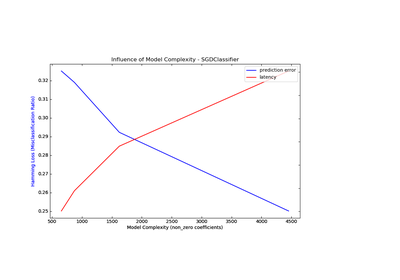Model Complexity Influence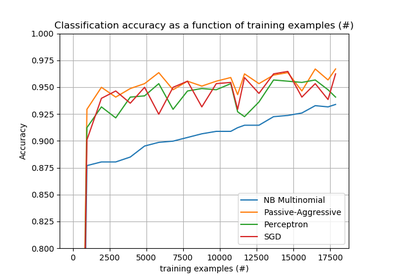Out-of-core classification of text documents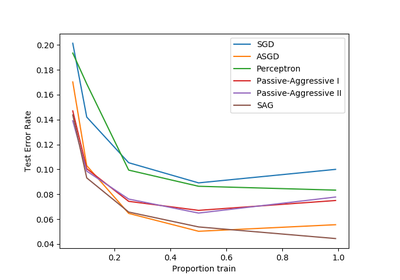Comparing various online solvers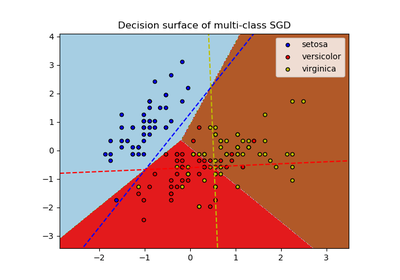Plot multi-class SGD on the iris dataset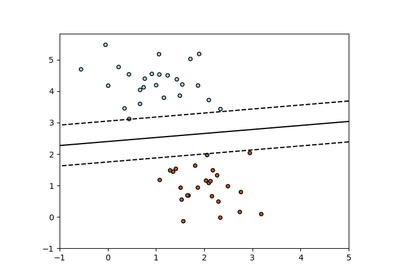SGD: Maximum margin separating hyperplane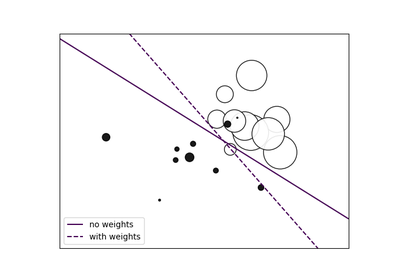SGD: Weighted samplesSample pipeline for text feature extraction and evaluationClassification of text documents using sparse features